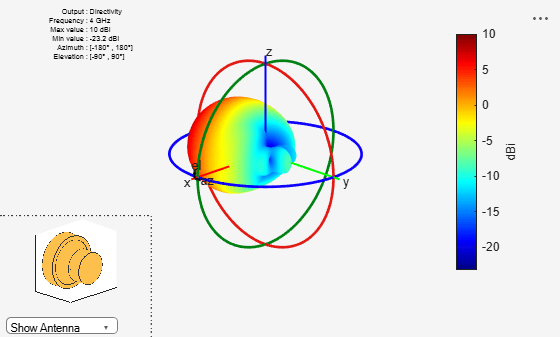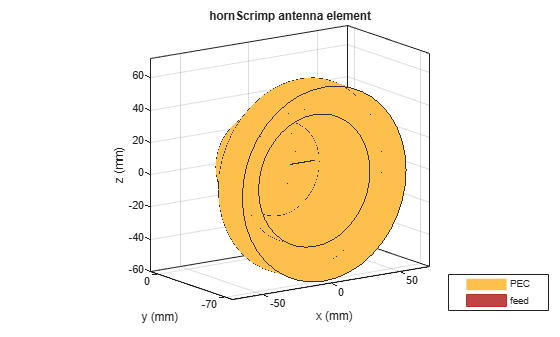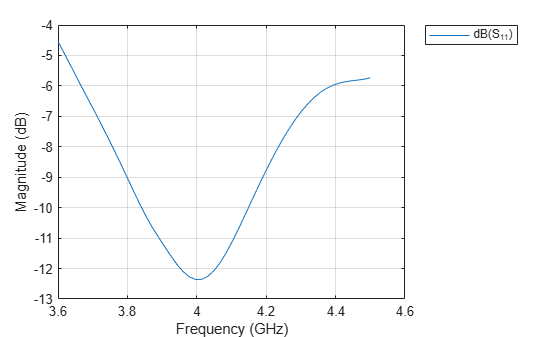# hornScrimp

Create Scrimp horn antenna

## Description

The `hornScrimp` object creates a Scrimp horn antenna. Scrimp (short circular ring loaded horn with minimized cross-polarization) horn antenna is a short, axially corrugated horn antenna with a single slot. Using this antenna provides high aperture efficiency, low cross-polarization, and low voltage standing wave ratio (VSWR) over a broad frequency band. These antennas are used for navigation satellite feeder links in a medium earth orbit (MEO).## Creation

### Syntax

``ant = hornScrimp``
``ant = hornScrimp(Name,Value)``

### Description

example

````ant = hornScrimp` creates Scrimp horn antenna with dimensions for an operating frequency of 4 GHz.```

example

````ant = hornScrimp(Name,Value)` sets Properties using one or more name-value pairs. For example, `hornScrimp('ConeHeight',0.05)` creates Scrimp horn antenna with the cone height of 0.05 meters.```

## Properties

expand all

Radius of the circular waveguide, specified as a positive scalar in meters.

Example: `'Radius',0.0760`

Example: `ant.Radius = 0.0760`

Data Types: `double`

Height of the circular waveguide, specified as a positive scalar in meters.

Example: `'WaveguideHeight',0.0340`

Example: `ant.WaveguideHeight = 0.0340`

Data Types: `double`

Height of the feed, specified as a positive scalar in meters.

Example: `'FeedHeight',0.0085`

Example: `ant.FeedHeight = 0.0085`

Data Types: `double`

Width of the feed, specified as a positive scalar in meters.

Example: `'FeedWidth',0.0200`

Example: `ant.FeedWidth = 0.0200`

Data Types: `double`

Signed distance along the waveguide height, specified as a real-valued scalar in meters.

Example: `'FeedOffset',0.03627`

Example: `ant.FeedOffset = 0.03627`

Data Types: `double`

Height of the cone, specified as a positive scalar in meters.

Example: `'ConeHeight',0.0540`

Example: `ant.ConeHeight = 0.0540`

Data Types: `double`

Radius of the cone, specified as a positive scalar in meters.

Example: `'ConeRadius',0.0540`

Example: `ant.ConeRadius = 0.0540`

Data Types: `double`

Radius of the cone aperture, specified as a positive scalar in meters.

Example: `'ApertureRadius',0.0760`

Example: `ant.ApertureRadius = 0.0760`

Data Types: `double`

Height of the cone aperture, specified as a positive scalar in meters.

Example: `'ApertureHeight',0.0760`

Example: `ant.ApertureHeight = 0.0760`

Data Types: `double`

Height of the stub, specified as a positive scalar in meters.

Example: `'StubHeight',0.0760`

Example: `ant.StubHeight = 0.0760`

Data Types: `double`

Type of the metal used as a conductor, specified as a metal material object. You can choose any metal from the `MetalCatalog` or specify a metal of your choice. For more information, see `metal`. For more information on metal conductor meshing, see Meshing.

Example: `m = metal('Copper'); 'Conductor',m`

Example: `m = metal('Copper'); ant.Conductor = m`

Tilt axis of the antenna, specified as:

• Three-element vector of Cartesian coordinates in meters. In this case, each coordinate in the vector starts at the origin and lies along the specified points on the X-, Y-, and Z-axes.

• Two points in space, each specified as three-element vectors of Cartesian coordinates. In this case, the antenna rotates around the line joining the two points in space.

• A string input describing simple rotations around one of the principal axes, 'X', 'Y', or 'Z'.

Example: `'TiltAxis',[0 1 0]`

Example: `'TiltAxis',[0 0 0;0 1 0]`

Example: `ant.TiltAxis = 'Z'`

Tilt angle of the antenna, specified as a scalar or vector with each element unit in degrees. For more information, see Rotate Antennas and Arrays.

Example: `'Tilt',90`

Example: `ant.Tilt = 90`

Example: `'Tilt',[90 90]`,```'TiltAxis',[0 1 0;0 1 1]``` tilts the antenna at 90 degrees about the two axes defined by the vectors.

Data Types: `double`

Lumped elements added to the antenna feed, specified as a `lumpedElement` object. You can add a load anywhere on the surface of the antenna. By default, the load is at the feed. For more information, see `lumpedElement`.

Example: `'Load',lumpedelement`, where `lumpedelement` is the load added to the antenna feed.

Example: `ant.Load = lumpedElement('Impedance',75)`

## Object Functions

 `show` Display antenna or array structure; display shape as filled patch `axialRatio` Axial ratio of antenna `beamwidth` Beamwidth of antenna `charge` Charge distribution on metal or dielectric antenna or array surface `current` Current distribution on metal or dielectric antenna or array surface `design` Design prototype antenna or arrays for resonance around specified frequency `efficiency` Radiation efficiency of antenna `EHfields` Electric and magnetic fields of antennas; Embedded electric and magnetic fields of antenna element in arrays `impedance` Input impedance of antenna; scan impedance of array `mesh` Mesh properties of metal or dielectric antenna or array structure `meshconfig` Change mesh mode of antenna structure `optimize` Optimize antenna or array using SADEA optimizer `pattern` Radiation pattern and phase of antenna or array; Embedded pattern of antenna element in array `patternAzimuth` Azimuth pattern of antenna or array `patternElevation` Elevation pattern of antenna or array `rcs` Calculate and plot radar cross section (RCS) of platform, antenna, or array `returnLoss` Return loss of antenna; scan return loss of array `sparameters` Calculate S-parameter for antenna and antenna array objects `vswr` Voltage standing wave ratio of antenna

## Examples

collapse all

Create Scrimp horn antenna with default properties.

`ant = hornScrimp`
```ant = hornScrimp with properties: Radius: 0.0292 WaveguideHeight: 0.0250 FeedHeight: 0.0175 FeedWidth: 3.0000e-04 FeedOffset: 0.0200 ConeHeight: 0.0362 ConeRadius: 0.0408 ApertureRadius: 0.0480 ApertureHeight: 0.0250 StubHeight: 0.0146 Conductor: [1×1 metal] Tilt: 0 TiltAxis: [1 0 0] Load: [1×1 lumpedElement] ```

View the antenna using the `show` function.

`show(ant)`Plot the radiation pattern of the Scrimp horn antenna at a frequency of 4 GHz.

`pattern(ant,4e9)`Create Scrimp horn antenna with the aperture radius of 0.06 meters.

```ant = hornScrimp; ant.ApertureRadius = 0.06;```

Visualize the antenna using the `show `function.

`show(ant)`Plot the S-parameters over a frequency range of 3.6 GHz to 4.5 GHz.

```s = sparameters(ant,linspace(3.6e9,4.5e9,51)); rfplot(s)```expand all

 Muhammad, S. A., A. Rolland, S. H. Dahlan, R. Sauleau, and H. Legay. “Hexagonal-Shaped Broadband Compact Scrimp Horn Antenna for Operation in C-Band.” IEEE Antennas and Wireless Propagation Letters 11 (2012): 842–45.

 Muhammad, S., A. Rolland, S. H. Dahlan, R. Sauleau and H. Legay. “Comparison Between Scrimp Horns and Stacked Fabry-Perot Cavity Antennas with Small Apertures.” 2012 6th European Conference on Antennas and Propagation (EUCAP) (2012): 817–820.